# 1. 状态空间描述

## 1.1. 输入-输出描述与状态空间描述的差异

1. 输入—输出描述(外部描述)

• 用传递函数、微分方程表征;
• 是系统的外部描述
• 是对系统的不完全描述
2. 状态空间描述(内部描述)

• 用状态空间表达式表征
• 是系统的内部描述
• 是对系统的完全描述

## 1.2. 状态、状态变量和状态向量

1. 状态

1. 状态变量

1. 状态向量

• 状态变量不是所有变量的总和
• 状态变量的选取不唯一
• 输出量可以选作状态变量
• 输入量不允许选作状态变量
• 状态变量有时是不可测量的
• 状态变量是时域的

## 1.3. 状态方程

$\dot{x}(t)=f[x(t),u(t),t]$

$x(t_{k+1})=f[x(t_k),u(t_k),t_k]$

## 1.4. 输出方程

$y(t)=g[x(t),u(t),t]$

$y(t_{k})=g[x(t_k),u_(t_k),t_k]$

## 1.5. 状态空间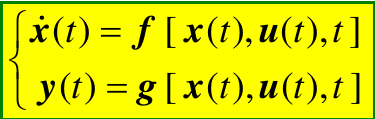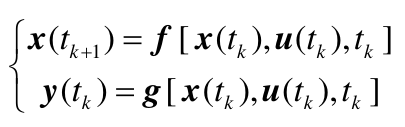## 1.6. 线性系统状态空间表达式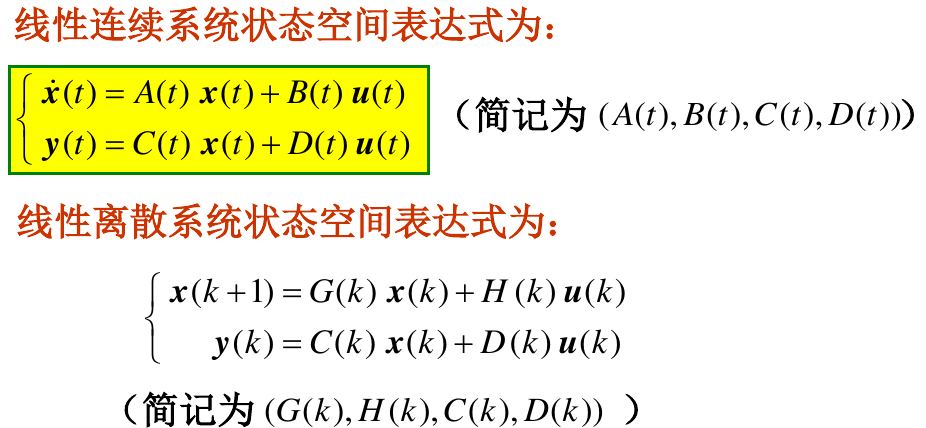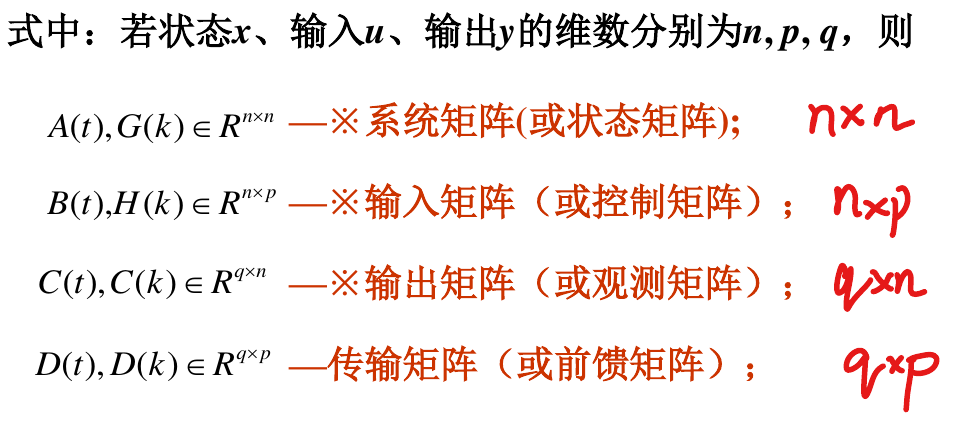## 1.7. 线性定常系统状态空间表达式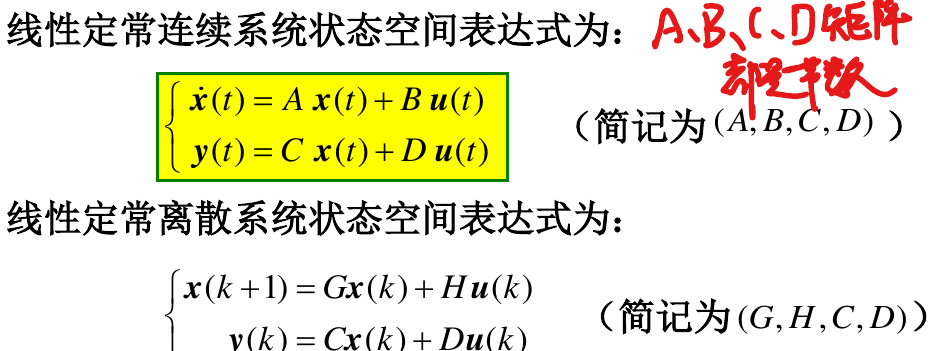## 1.8. 线性系统和非线性系统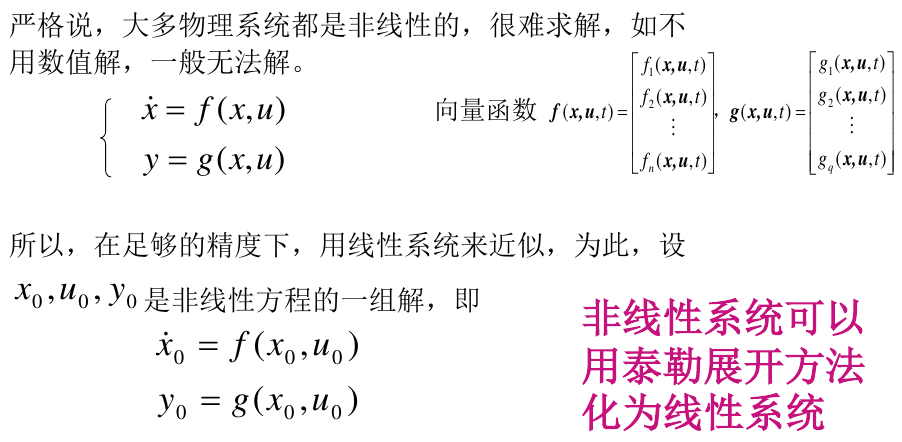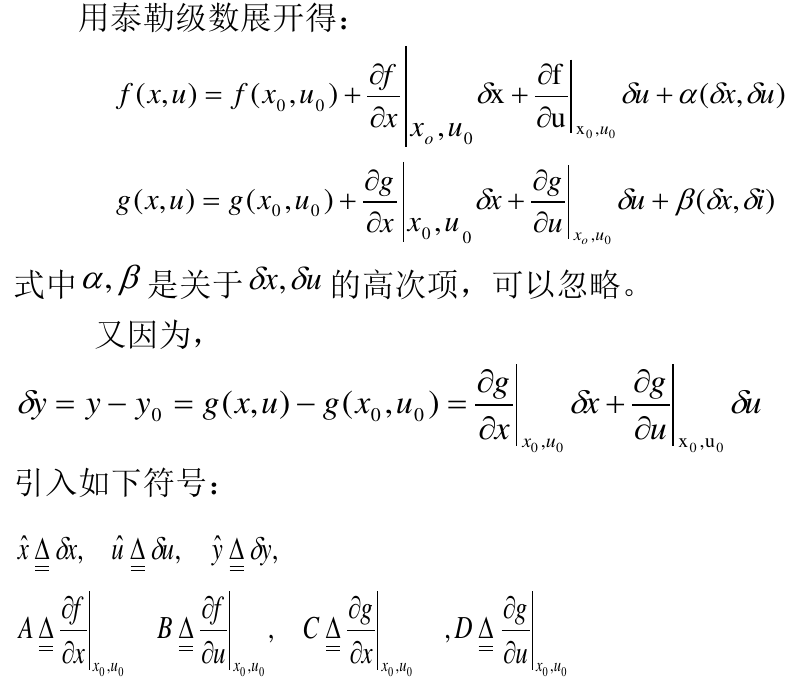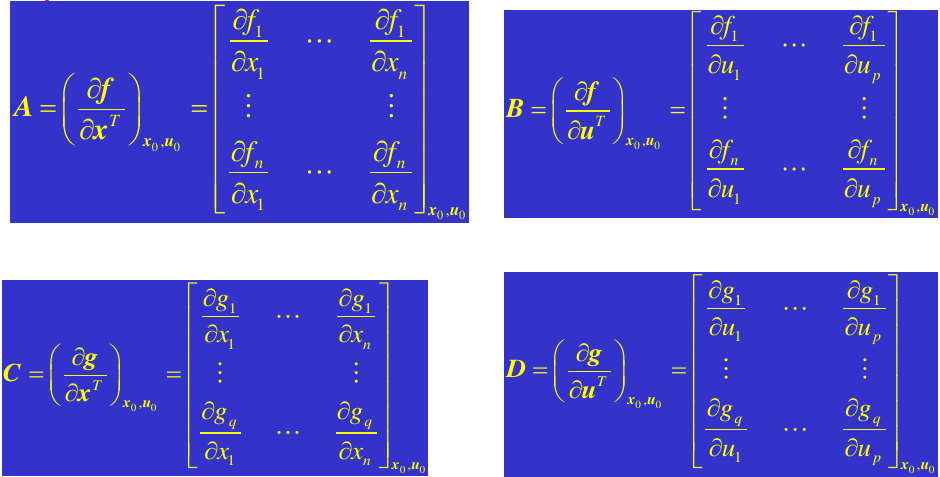# 2. 传递函数导出状态空间描述

## 2.1. 给定单输入,单输出线性时不变系统的输入输出描述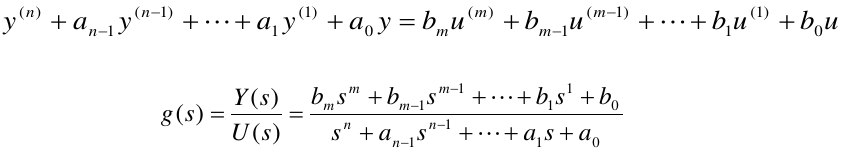(1)m<n，系统为严真情形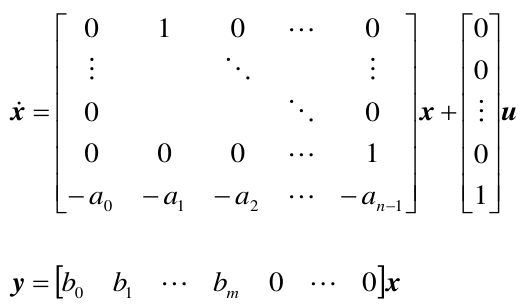(2)m=n，系统为真情形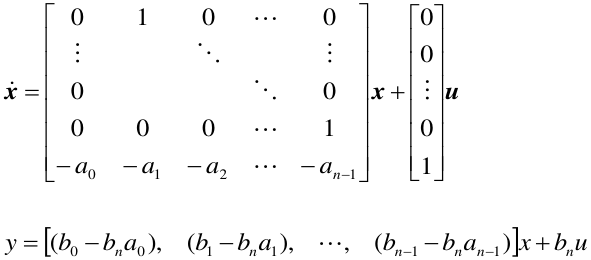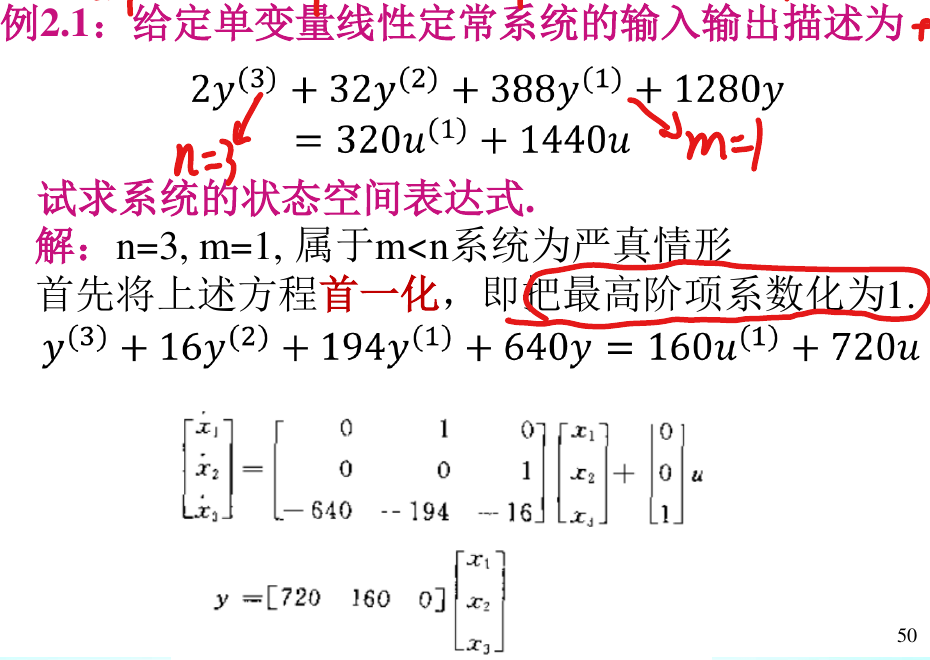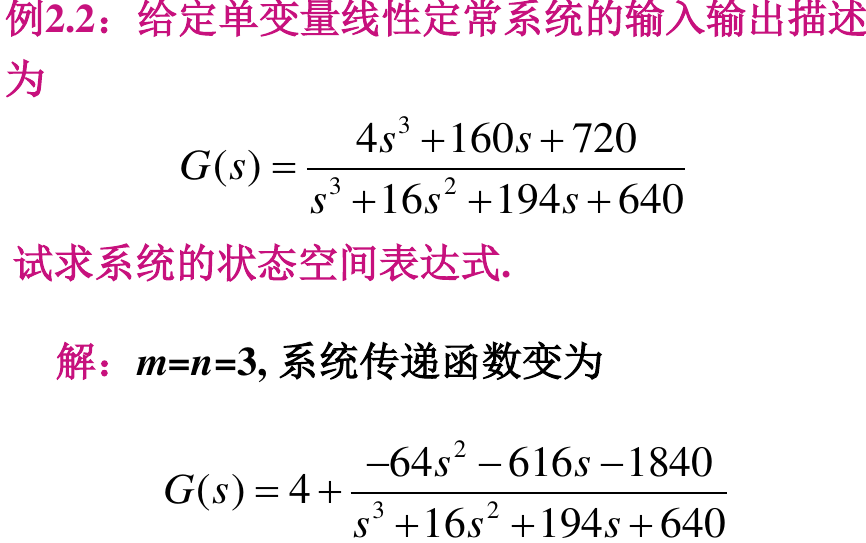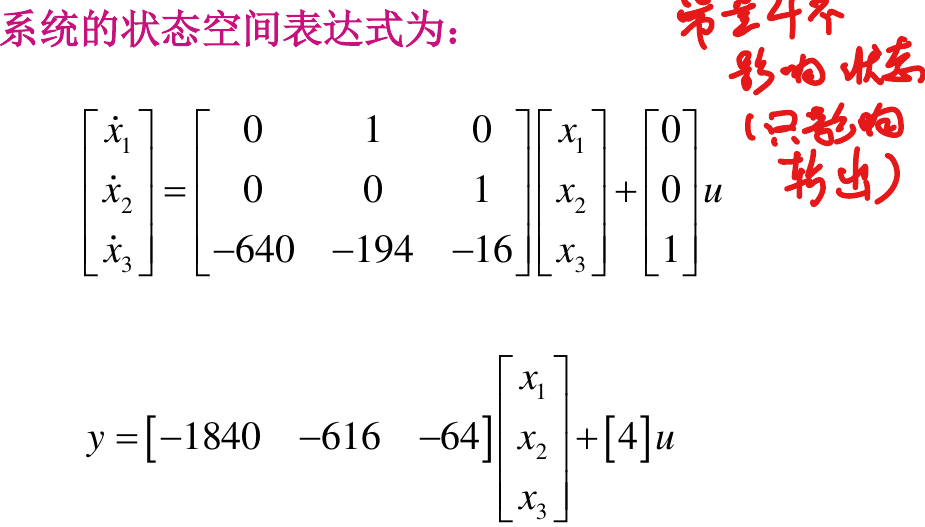## 2.2. 给定单输入,单输出线性时不变系统的输入输出描述

(1)m=0情形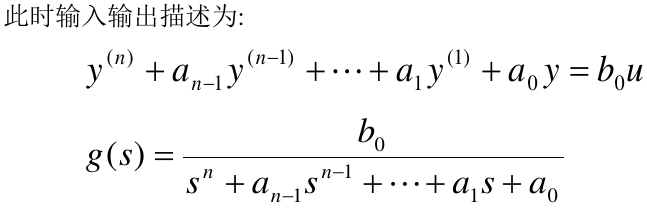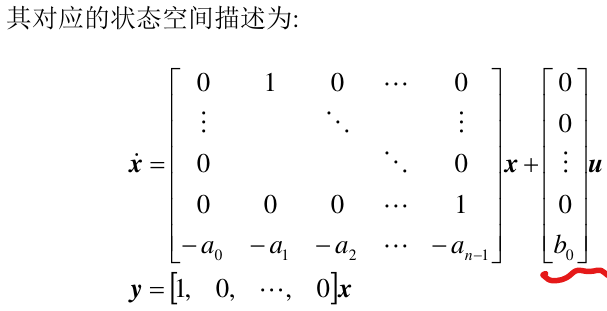(2)m≠0情形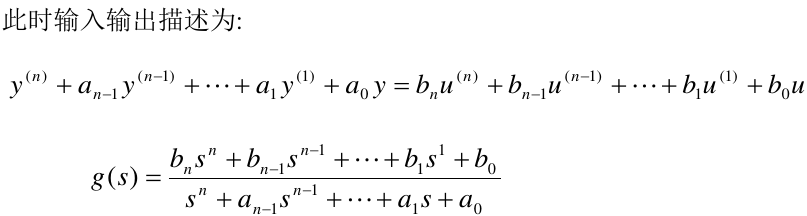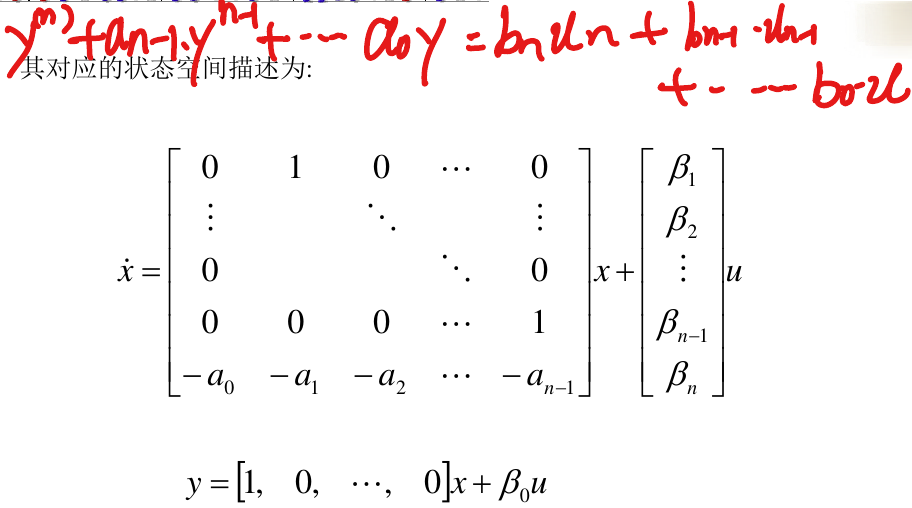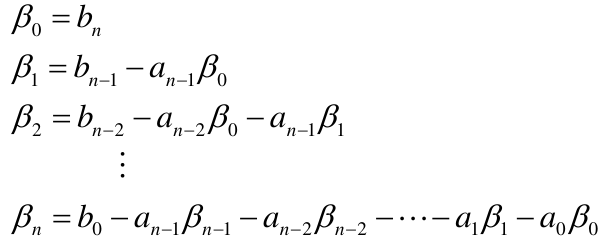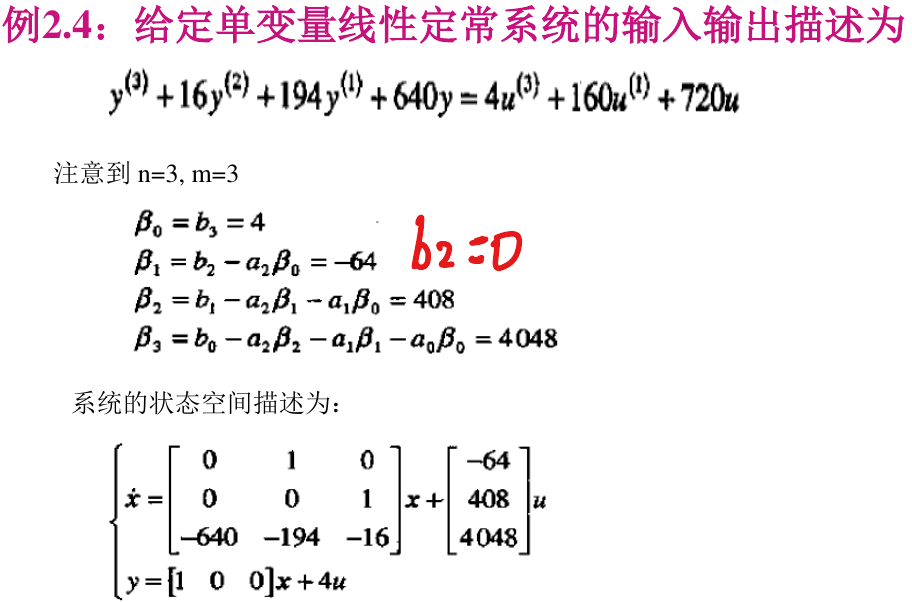## 2.3. 给定单输入,单输出线性时不变系统的传递函数描述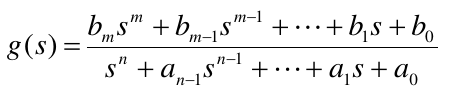(1)m<n，系统为严真情形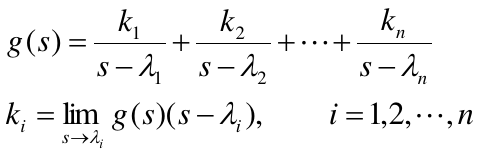\begin{aligned} \dot{x}= \begin{bmatrix} \lambda_1, &~ & ~&~ \\ ~ & \lambda_1 & ~ &~ \\ ~ & ~ & \ddots & ~ \\ ~ & ~ & ~ & \lambda_n \end{bmatrix} x + \begin{bmatrix} k_1 \\ k_2 \\ \vdots \\ k_n \end{bmatrix} u \end{aligned}

$y=[1,1,\cdots,1]x$

(1)m=n，系统为真情形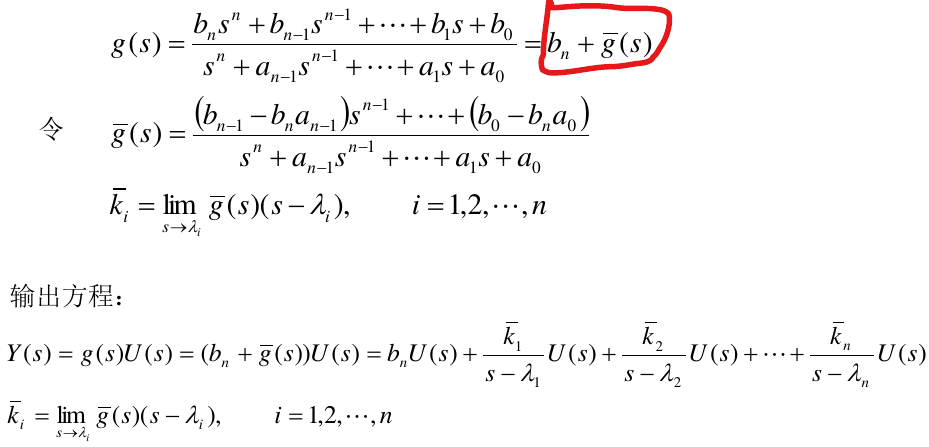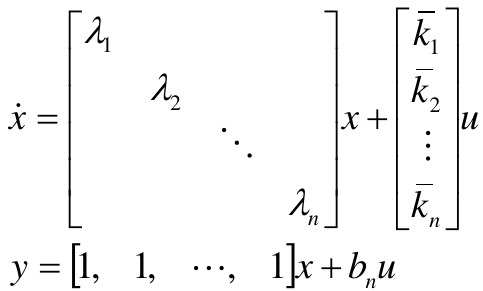## 2.4. 由方框图描述导出状态空间描述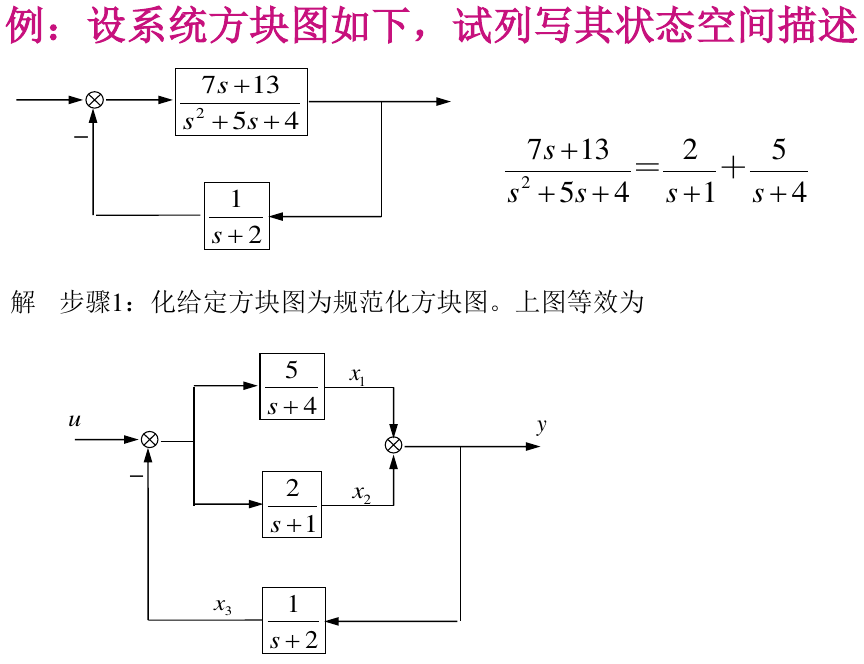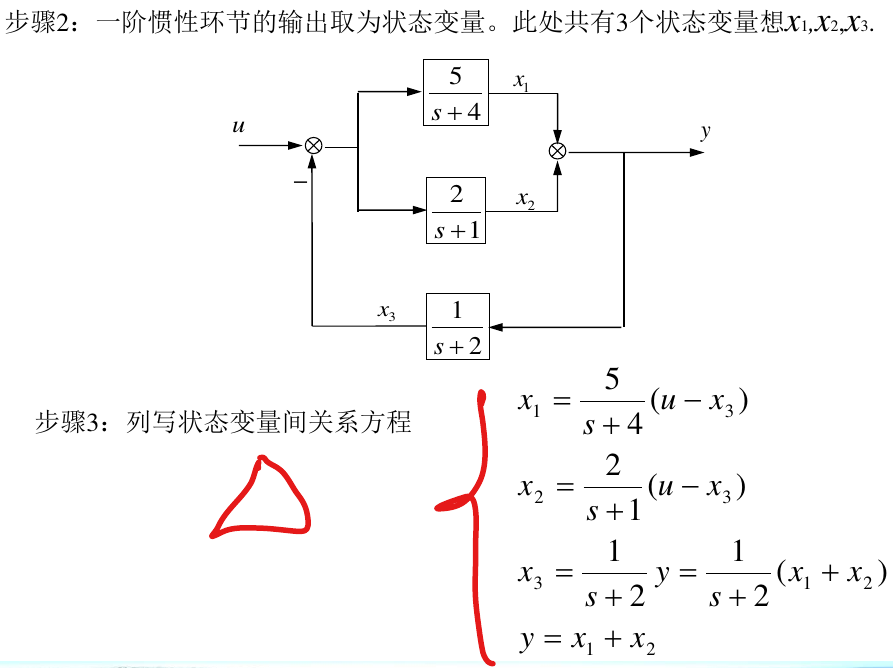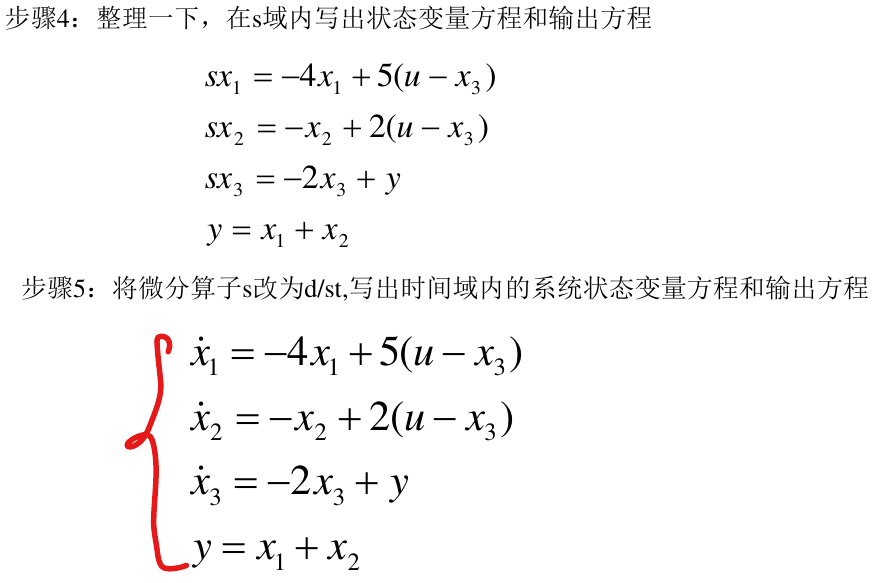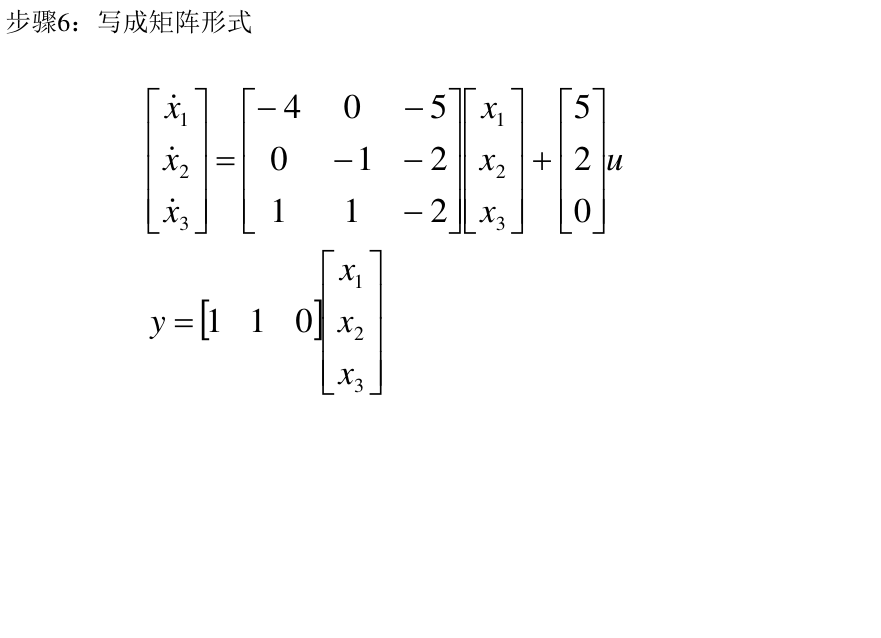# 3. 状态空间描述化标准型、约旦型

## 3.1. 前提基础

### 3.1.1. 特征多项式

• 特征矩阵： $$(sI-A)$$
• 预解矩阵: $$(sI-A)^{-1}$$
• 特征多项式: $$\det(sI-A)$$

(1)特征多项式

$\alpha(s)=\det(sI-A)=s^n + \alpha_{n-1}s^{n-1}+\cdots + \alpha_1 s + \alpha_0$

(2)凯莱哈密顿定理

$\alpha(A)=A^n+ \alpha_{n-1}A^{n-1}+ \cdots + \alpha_1 A + \alpha_0 I =0$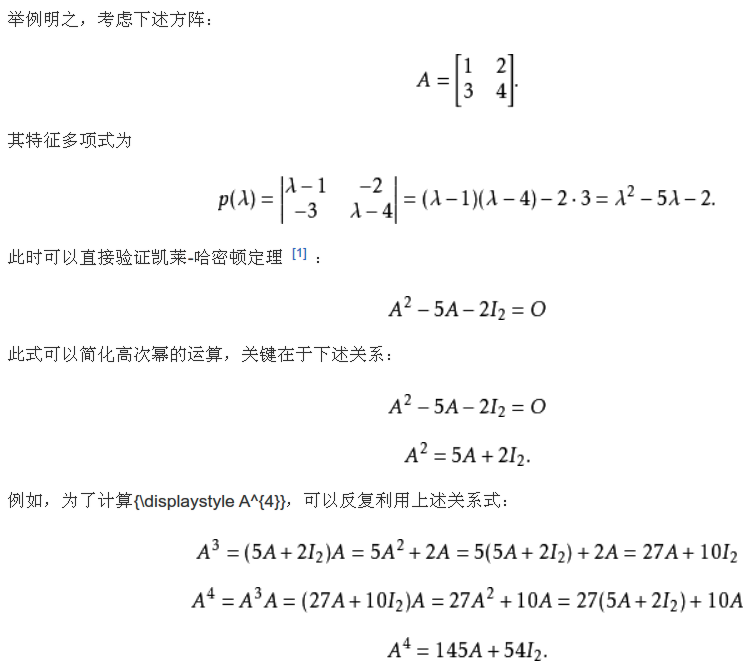(3)莱弗勒算法——计算特征多项式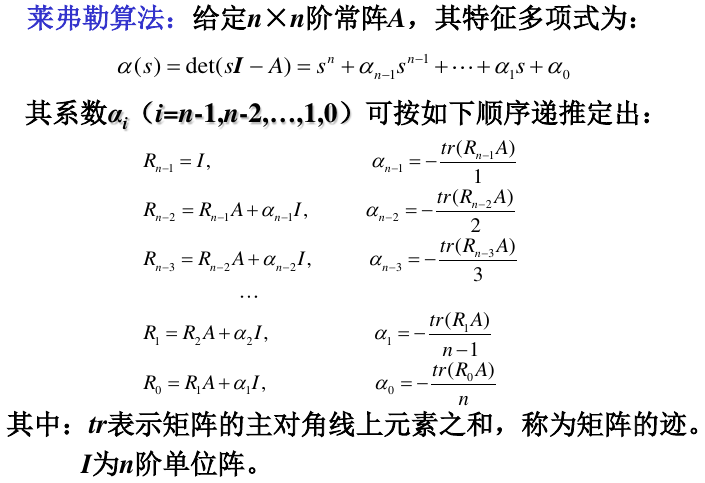• 通过迭代的方式，求出大常数矩阵A的特征多项式$$(sI-A)$$

### 3.1.2. 特征值和特征向量

$\dot{x}=Ax+Bu$

$\det(sI-A)=0$

\begin{aligned} \lambda_i v_i = A v_i \\ \Longrightarrow (\lambda_i I -A)v_i = 0 \end{aligned}

### 3.1.3. 特征值的代数重数和几何重数

(1)代数重数$$\sigma_i$$

(2)几何重数$$\alpha_i$$

即将特征值代入上面齐次方程组基础解系的解向量个数

$\alpha_i=n- rank(\lambda_i I -A)$

• 单特征值只对应一个特征向量(即$$\alpha_i=1$$)
• 有重根的特征值，可能对应多个独立的特征向量

## 3.2. 标准型

### 3.2.1. 特征值两两互异(没有重根)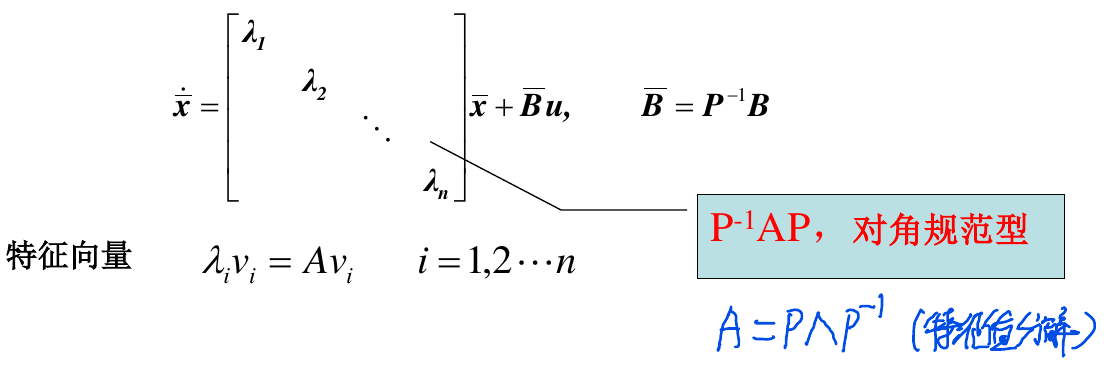• 对A矩阵进行特征值分解$$A=P \Lambda P^{-1}$$
• 那么，可得到化为标准型之后的$$\bar{A},\bar{B}$$
• $$\bar{A}=P^{-1}AP$$
• $$\bar{B}=p^{-1}B$$
• 状态方程可重写为$$\dot{x}=\bar{A}x+\bar{B}u$$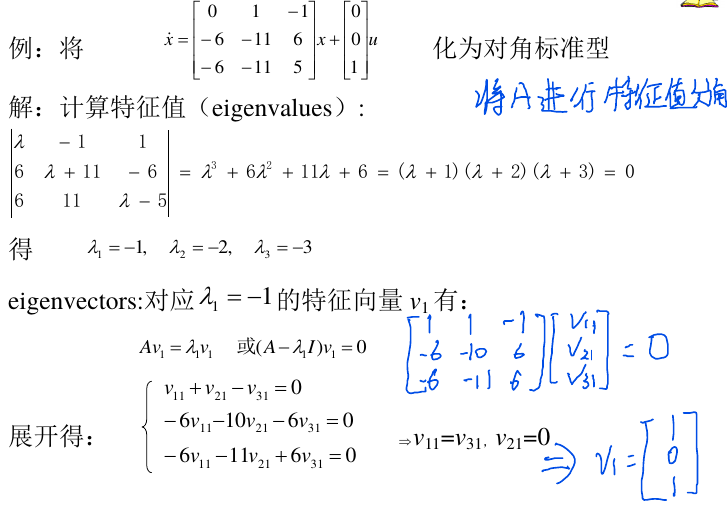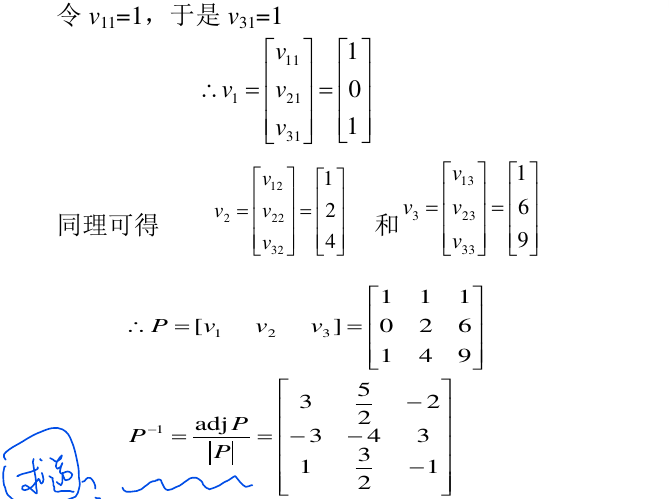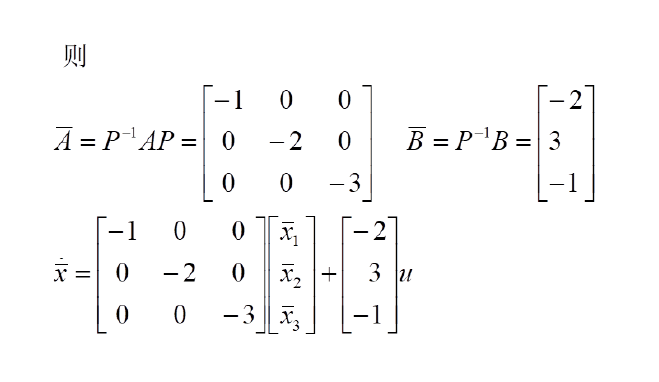(特例)友矩阵形式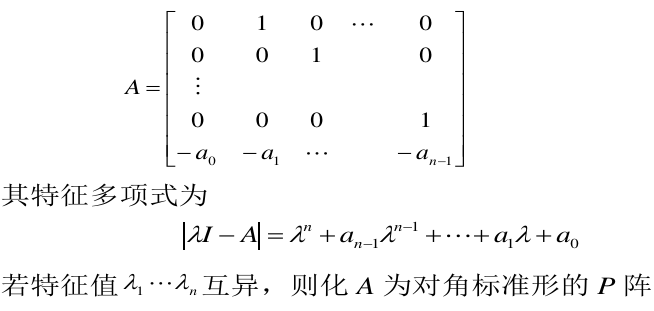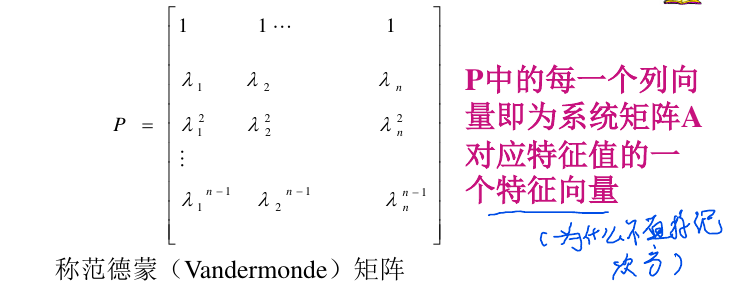### 3.2.2. 特征值有重根(但是仍然有n个独立特征向量)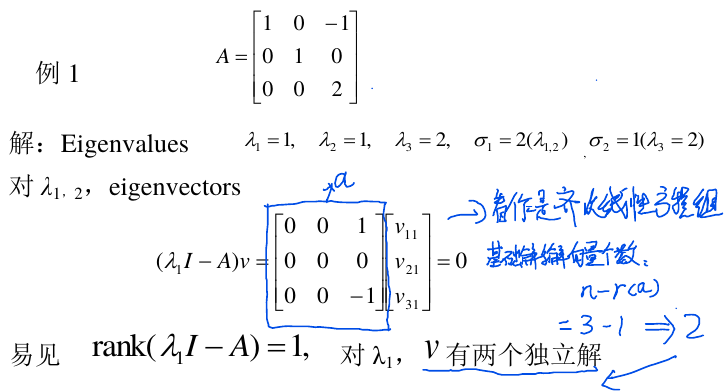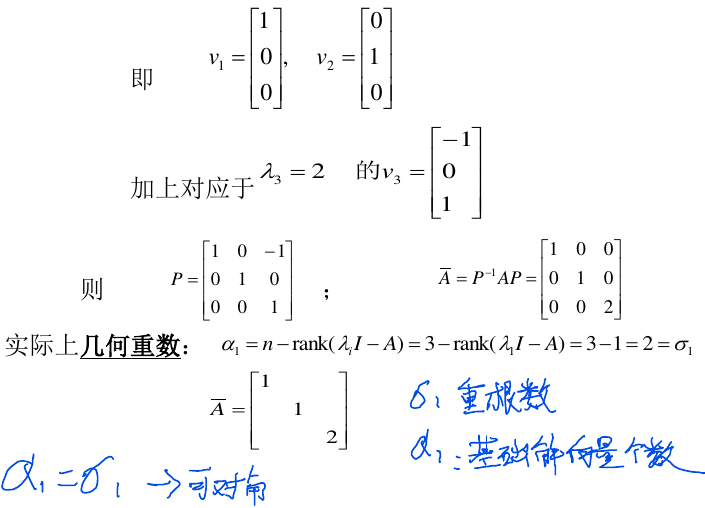• 系统矩阵A的n个特征值两两互异
• 系统矩阵A有重特征值，且所有特征值的几何重数都等于其代数重数

## 3.3. 约当规范形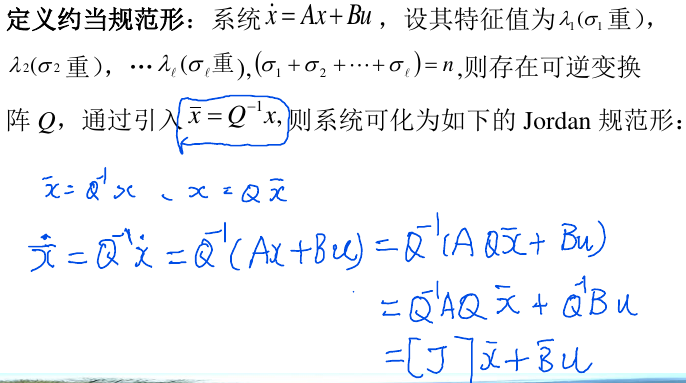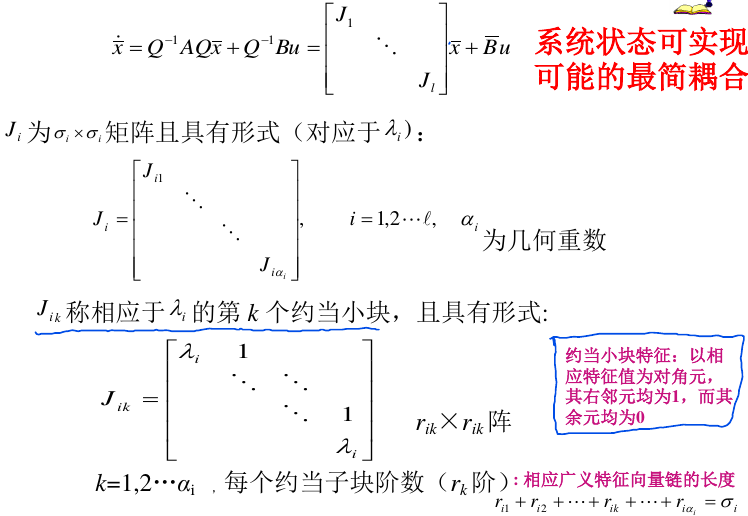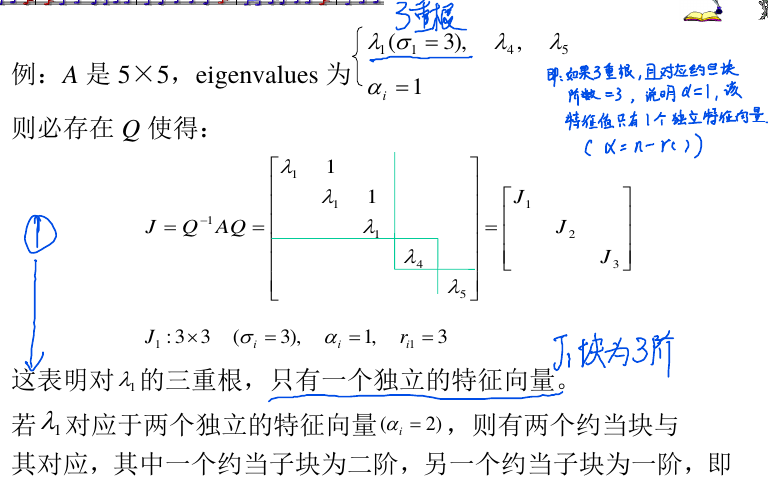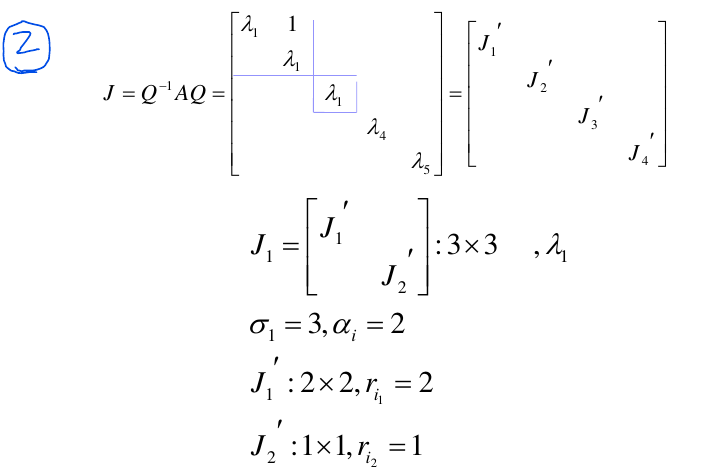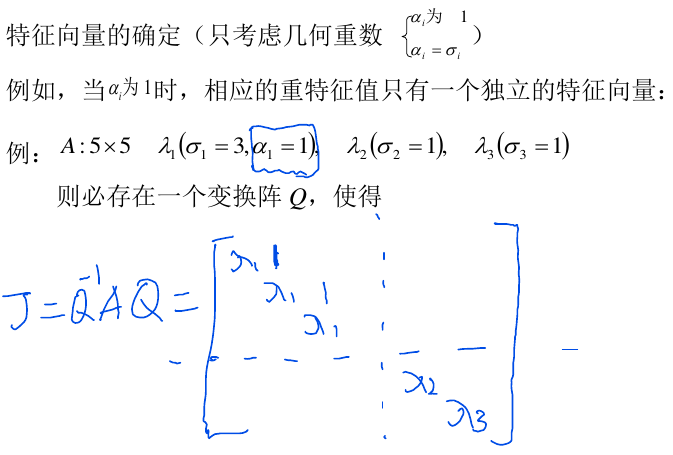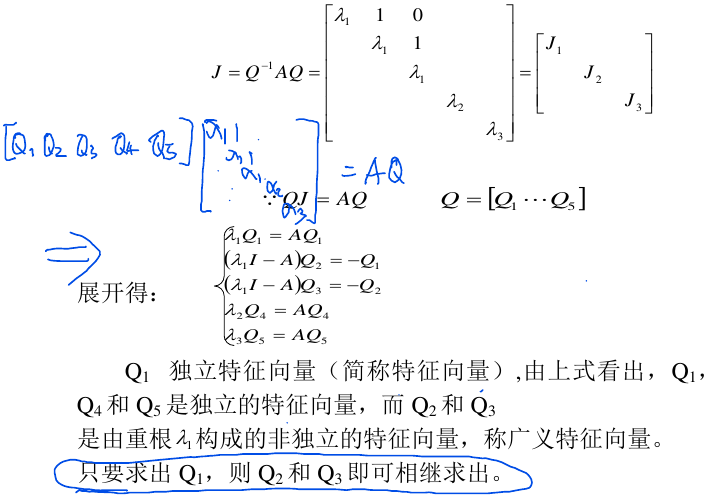(特例)友矩阵形式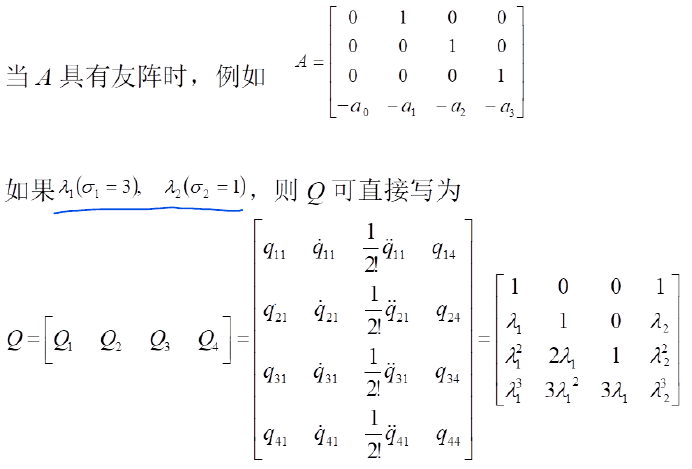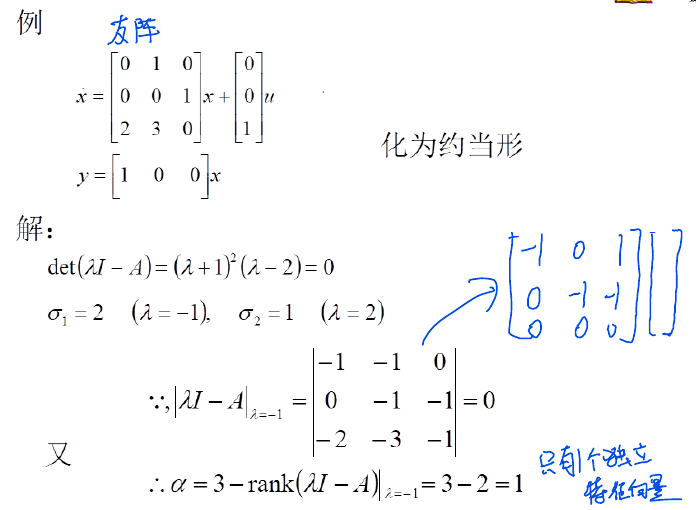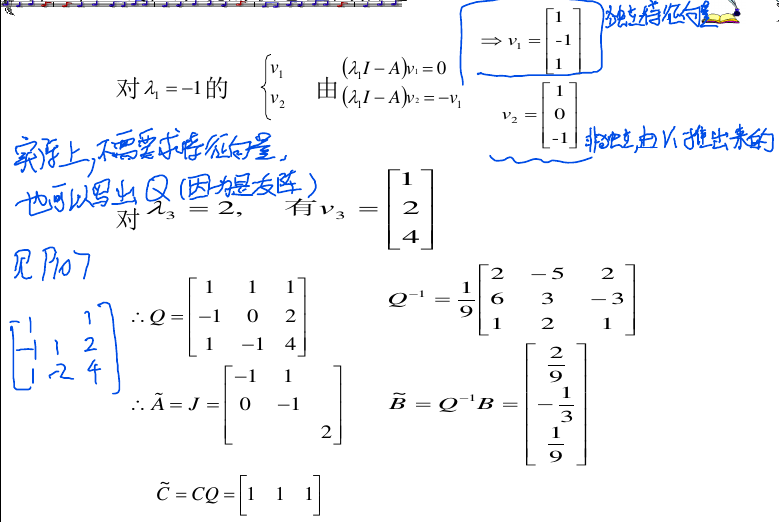# 4. 状态空间===>传递函数矩阵

## 4.1. 传递函数矩阵

1. 单输入单输出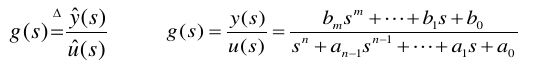1. 多输入多输出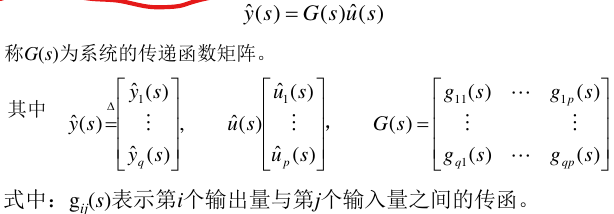## 4.2. 传递函数严真性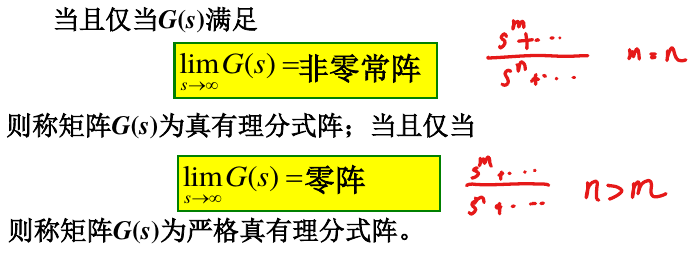## 4.3. ABCD矩阵===>传递函数(重点在这里)

\begin{aligned} \dot{x}=Ax+Bu \\ y=Cx+Du \\ \\ \Longrightarrow G(s)=C(sI-A)^{-1}B+D \end{aligned}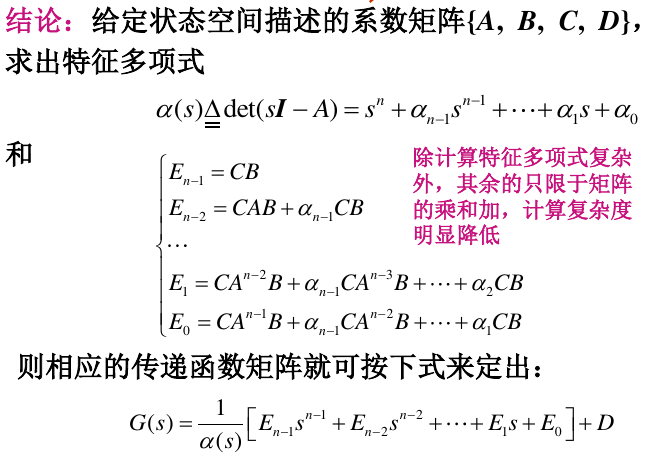# 5. 关于代数等价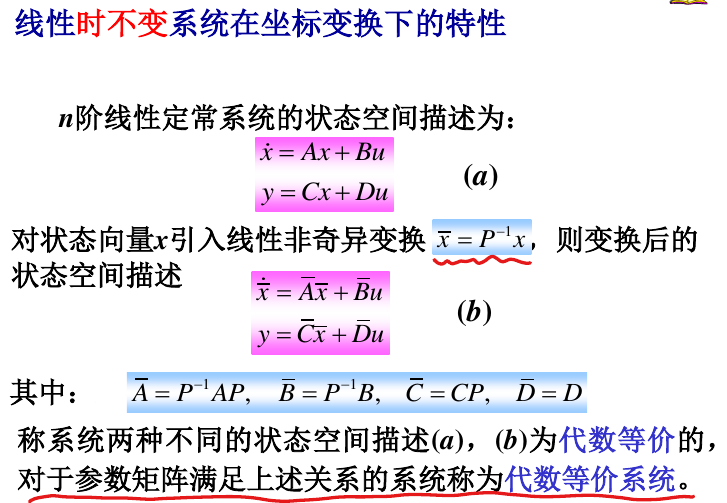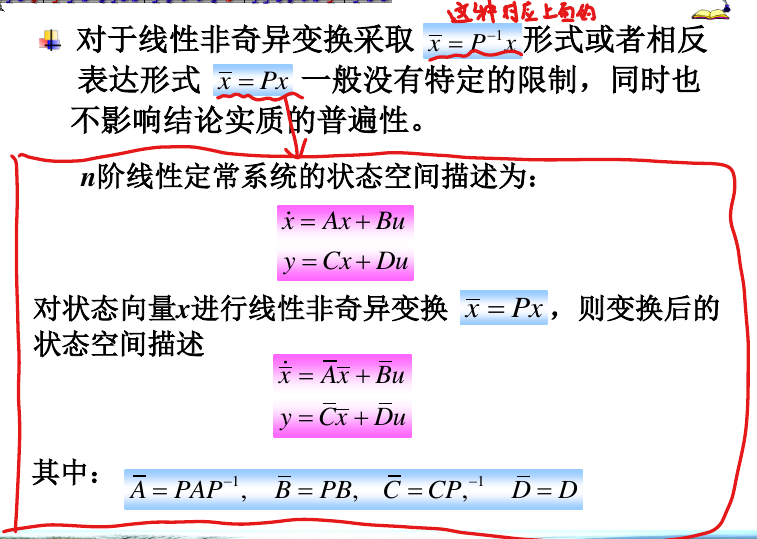• 两个系统为代数等价的必要条件是它们具有相同的维数
• 同一系统采用不同的状态变量组所导出的两个状态空间描述之间,必然是代数等价的
• 代数等价系统具有相同的传递函数矩阵,但相反的情况却不一定成立
• 代数等价系统具有相同的特征值